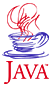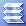Home : Course Map : Chapter 6 : Java : Drawing PolygonsIntroduction AWT Swing Containers   Demo 1 UI Components   Demo 2 UI Layout   Demo 3   Demo 4 Text Display   Demo 5 Drawing   Demo 6   Demo 7 Draw Polygons   Demo 8   Demo 9 Colors Text Draw    Demo 10 Images   Demo 11 Exercises
 Supplements AWT   Demo 1 Drawing   Demo 2 Text Drawing   Demo 3 UI Components   Demo 4 Java2D Shapes & Areas   Demo 1   Demo 2 Stroke & Paint   Demo 3 Transforms   Demo 4 Gradients&Textures   Demo 5   Demo 6 Text   Demo 7   Demo 8
 About JavaTech      Codes List      Exercises      Feedback      References      Resources      Tips      Topic Index      Course Guide      What's New

In the examples up till now, we have used methods like drawOval() or drawRect() to draw these common geometric forms.

One approach is to use multiple calls to drawLine() to draw a sequence of straight line segments that outlines the desired shape.

But a faster approach is instead to use a single call to drawPolyline(). This will speed up the drawing since the process needs only one invocation of the method, rather than one for each line segment.

import javax.swing.*;
import java.awt.*;

/** Example demonstrating drawPolyline().*/
public class Polygon1Applet extends JApplet
{
public void init ()  {
Container content_pane = getContentPane ();

int width = getSize ().width;
int height= getSize ().height;

int num_points = 21;

// Create an instance of DrawingPanel
Polygon1Panel polygon1_panel =
new Polygon1Panel (width,height,num_points);

// Add the DrawingPanel to the contentPane.

}
}

/** Draw a polygon with drawPolyline() on
* this JPanel subclass. **/
class Polygon1Panel extends JPanel
{
int fWidth,fHeight;
int fNumPoints;
double fFactor;

Polygon1Panel (int width, int height, int nPoints) {
fNumPoints = nPoints;
fWidth = width;
fHeight= height;
fFactor = 2.0 * Math.PI / fWidth;
} // ctor

public void paintComponent (Graphics g) {
// First paint background unless you will
// paint whole area yourself.
super.paintComponent (g);

// Create arrays of points for each
// segment of the polygon
int [] x = new int[fNumPoints];
int [] y = new int[fNumPoints];

// Select horizontal step size
double x_del=  ((double)fWidth)/ (fNumPoints-1);

// Find coordinates of the display center
int x_offset = fWidth/2;
int y_offset = fHeight/2;

// Choose amplitude for the sine curve
int amp =  (int) (y_offset * 0.9);

// Create a sine curve from a sequence
// of short line segments
for  (int i=0; i < fNumPoints; i++) {
x[i] =  (int) (i * x_del);
y[i] =  (int) (amp * Math.sin (fFactor * x[i]) )
+ y_offset;
}

// Set the line color to red
g.setColor (Color.red);

// Draw curve with single call to drawPolyline
g.drawPolyline (x,y,fNumPoints);

// Change the line color and draw the x-y axes
g.setColor (Color.green);
g.drawLine (0,y_offset,fWidth-1,y_offset);
g.drawLine (x_offset,0,x_offset,fHeight-1);

} // paintComponent

} // class Polygon1Panel

The method

drawPolygon(int[] x, int[] y, int nPoints)

will work similarly, except it will connect the last point to the first if they are not the identical.

An interesting overriden version of this method

drawPolygon(Polygon poly)

uses an instance of the Polygon class to hold the points.

import javax.swing.*;
import java.awt.*;

/** Example demonstrating drawPolygon() method. */
public class Polygon2Applet extends JApplet
{
public void init()  {
Container content_pane = getContentPane();

int width = getSize().width;
int height= getSize().height;

int num_points = 21;

// Create an instance of DrawingPanel
Polygon2Panel polygon2_panel =
new Polygon2Panel(width,height,num_points);

// Add the DrawingPanel to the contentPane.

} // init
} // class Polygon2Applet

/** Draw a polygon with drawPolygon() on
* this JPanel subclass. **/
class Polygon2Panel extends JPanel
{
int fWidth,fHeight;
int fNumPoints;
double fFactor;

Polygon2Panel (int width, int height, int numPoints)  {
fNumPoints = numPoints;
fWidth = width;
fHeight= height;
fFactor = 2.0 * Math.PI / (fNumPoints-1);
} // ctor

public void paintComponent(Graphics g) {
// First paint background
super.paintComponent(g);

// Create arrays of points for each
// segment of the polygon
int [] x = new int[fNumPoints];
int [] y = new int[fNumPoints];

// Find coordinates of the display center
int x_offset = fWidth/2;
int y_offset = fHeight/2;

// Choose radius for the circle
int radius = (int)(y_offset * 0.9);

// Create a sine curve from a sequence
// of short line segments
for (int i=0; i < fNumPoints; i++)
{
x[i] = (int)( radius * Math.cos(fFactor * i) )
+ x_offset;
y[i] = (int)( radius * Math.sin(fFactor * i) )
+ y_offset;
}

// Set the line color to red
g.setColor(Color.red);

// Create a polygon object from these point arrays
Polygon poly = new Polygon(x,y,fNumPoints);

// Then pass the polygon object for drawing
g.drawPolygon(poly);

// Change the line color and draw the x-y axes
g.setColor(Color.green);
g.drawLine(0,y_offset,fWidth-1,y_offset);
g.drawLine(x_offset,0,x_offset,fHeight-1);

} // paintComponent

} // class Polygon2Panel

This graphics technique - creating a shape object, here a polygon, and then telling it to draw itself - is a key feature of the Java2D approach to graphics that we will briefly discuss in Chapter 6: Java : Supplements.

The Polygon class also has several interesting methods such as

public boolean contains(int x, int y);

that indicates if the given point is within the polygon and

public void translate(int dx, int dy);

that adds dx and dy to each of the points of the polygon and thus move the shape by that amount.

Latest update: Oct. 26, 2004

 Tech Java Tech Graphics Starting to Plot   Demo 1 Drawing Panel   Demo 2 Histogram Display   Demo 3 Exercises
 Physics Display Text Data   Demo 1 Plot Data   Demo 2 Find Max/Min   Demo 3 ExercisesPart I Part II Part III Java Core 1  2  3  4  5  6  7  8  9  10  11  12 13 14 15 16 17 18 19 20 21 22 23 24 Supplements 1  2  3  4  5  6  7  8  9  10  11  12 Tech 1  2  3  4  5  6  7  8  9  10  11  12 Physics 1  2  3  4  5  6  7  8  9  10  11  12

Java is a trademark of Sun Microsystems, Inc.